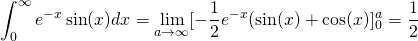# Solution assignment 05 Improper integrals

### Assignment 5

Calculate: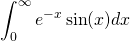### Solution

It is clear that this integral converges, because the function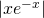is greater than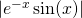from some value of. The integral of the first function yields a finite result (converges) and this will be the case with the second function. See the graph of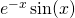in the figure.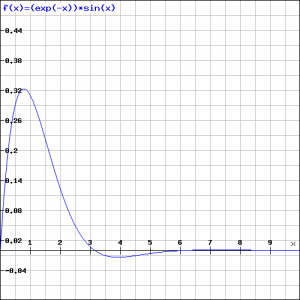The integral has a finite value.

We first look at the calculation of the indefinite integral and use integration by parts: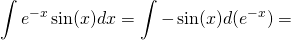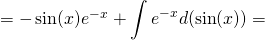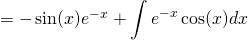It seems that this has not helped us a lot, but by applying integration by parts again we get the following result: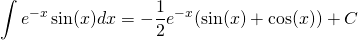Verify this result by differentiation.

Now we get: